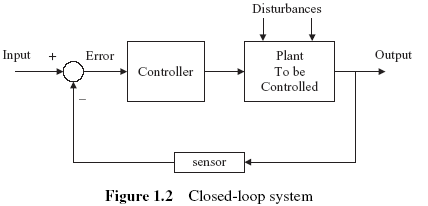Home | Gadget | Fuzzy | Lecturer  | Power  | Transceiver Find more type here... THE IDEA OF SYSTEM CONTROL by D.Ibrahim     Control engineering is concerned with controlling a dynamic system or plant. A dynamic system can be a mechanical system, an electrical system, a fluid system, a thermal system, or a combination of two or more types of system. The behaviour of a dynamic system is described by differential equations. Given the model (differential equation), the inputs and the initial conditions, we can easily calculate the system output.   A plant can have one or more inputs and one or more outputs. Generally a plant is a continuous-time system where the inputs and outputs are also continuous in time. For example, an electromagnetic motor is a continuous-time plant whose input (current or voltage) and output (rotation) are also continuous signals. A control engineer manipulates the input variables and shapes the response of a plant in an attempt to influence the output variables such that a required response can be obtained.   A plant is an open-loop system where inputs are applied to drive the outputs. For example, a voltage is applied to a motor to cause it to rotate. In an open-loop system there is no knowledge of the system output. The motor is expected to rotate when a voltage is applied across its terminals, but we do not know by how much it rotates since there is no knowledge about the output of the system. If the motor shaft is loaded and the motor slows down there is no knowledge about this. A plant may also have disturbances affecting its behaviour and in an open-loop system there is no way to know, or to minimize these disturbances.Figure 1.1 shows an open-loop system where the system input is expected to drive the system output to a known point (e.g. to rotate the motor shaft at a specified rate). This is a single-input, single-output (SISO) system, since there is only one input and also only one output is available. In general, systems can have multiple inputs and multiple outputs (MIMO). Because of the unknowns in the system model and the effects of external disturbances the open-loop control is not attractive. There is a better way to control the system, and this is by using a sensor to measure the output and then comparing this output with what we would like to see at the system output. The difference between the desired output value and the actual output value is called the error signal. The error signal is used to force the system output to a point such that the desired output value and the actual output value are equal. This is termed closed loop control, or feedback control.Figure 1.2 shows a typical closed-loop system. One of the advantages of closed-loop control is the ability to compensate for disturbances and yield the correct output even in the presence of disturbances. A controller (or a compensator) is usually employed to read the error signal and drive the plant in such a way that the error tends to zero. This material comes from any source reference and the copyright is own the author him self not this sites Copyright 2009 (c) technosains.com  All Rights Reserved